# Impedance Matching Fundamentals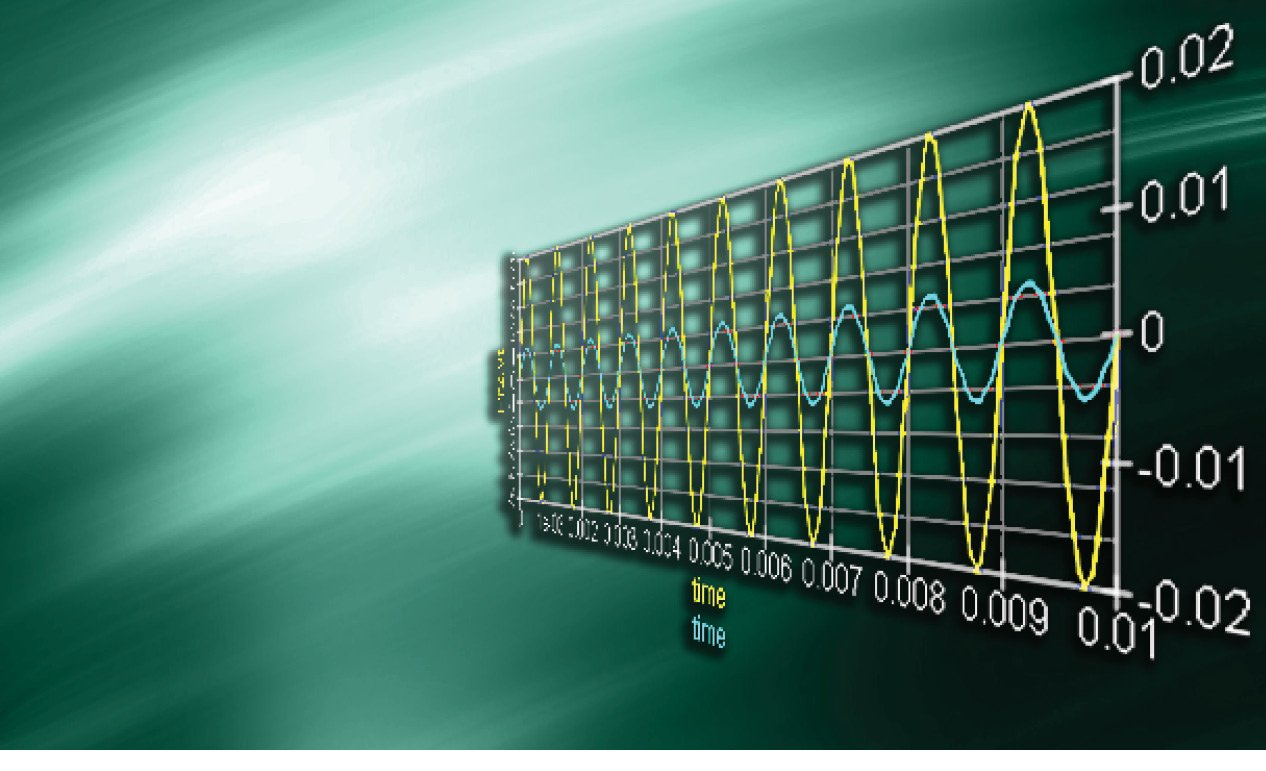Written by

### Robert had to troubleshoot an impedance matching issue for a customer. That experience was the impetus for him to dig deeper into the subject and to write this primer on impedance matching fundamentals.

Welcome back to the Darker Side. Albert Einstein claimed: “Most of the fundamental ideas of science are essentially simple, and may, as a rule, be expressed in a language comprehensible to everyone.” I’m not sure this is still the case with 21st-century physics (just try to find someone who can explain superstring theory in simple terms). Nevertheless, it is also my credo for this column. I try to present complex concepts in simple terms. In that spirit, let’s tackle a tough subject.

During the last 10 years, I’ve covered the topic of impedance matching several times. Once again, this will be my subject this month. Why? Simply because it is often misunderstood—or considered to be black magic. It isn’t. Impedance matching is both simple and fundamental. Moreover, it is not only a concern for radio frequency or ultra-high speed designers. It can happen close to direct current (DC) too. Do you want a proof? Just one month ago, one of our customers came to us with an efficiency issue on a specific switching power supply. And guess what. It was an impedance mismatch issue. We added a small inductor to the proper location and gained another happy customer.

Take a seat. In this article, I’ll remind you about the basics of impedance matching. I will not cover anything other than audio frequencies, so you’ll be able to easily reproduce and test my examples.

##### THE BASICS

Let’s start with the most basic example: DC. Suppose that you have a battery with a voltage U0, say, 12 V. Unfortunately, a perfect source doesn’t exist, so this battery has an internal parasitic serial resistance (RIN) of 1 Ω. This means that the battery voltage is U0 = 12 V with no load. It’s only 11 V with a 1-A load, etc. Assume that you use this battery as a source and connect its terminals on an external resistor, R (see Figure 1). The application of Ohm’s law is then straightforward. The current through the circuit is:

The power dissipated in the external resistor, R, is easily calculated: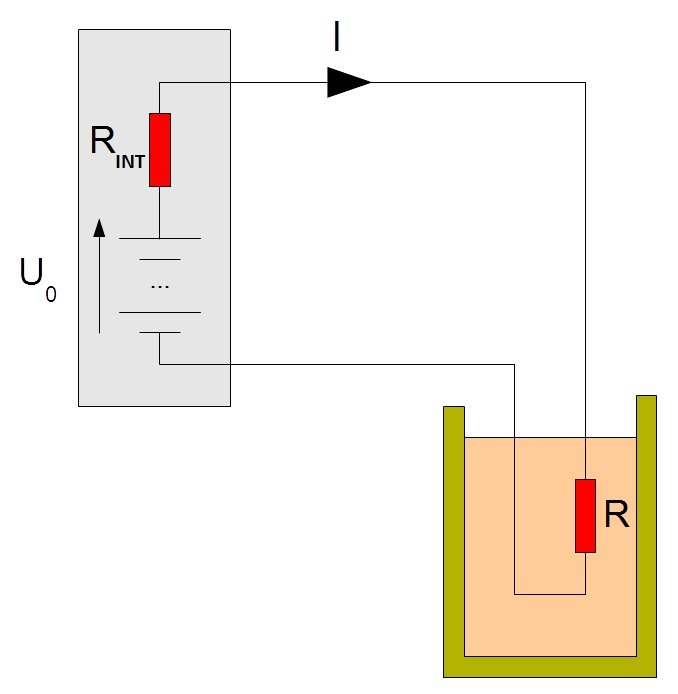FIGURE 1 This is the simplest impedance matching example with a DC source. What is the value of the load R for a maximum dissipated power in this load?

Now imagine that you can’t change the source internal resistance, RIN, but want to dissipate as much power as possible on the resistor R. This means you want to transfer as much energy as possible from the source to the load. What are your choices? If you use a high value for R, then the current going through it will be small, so the dissipated power will be low. Conversely, if you use a very low-value resistor (close to a short circuit), then the current through the circuit will be very high, but the power dissipated in your resistor will be very low too. In fact, in the latter case, a lot of power will be dissipated, but only in the battery’s internal resistance and not in the external resistor. In Equation 2, P is zero with either R = 0 or R = infinite. So, there must be an intermediate value for R that gives a maximum power dissipation in the external resistor, meaning an optimal power transfer from the battery to the load. This is impedance matching, and the optimal value is R = RIN. You can check it by yourself by either plotting the function R/(RIN + R)2 with RIN = 1 or checking if its derivative is null for R = RIN (that is if you remember how to calculate a derivative).

In a nutshell, the optimal load for extracting as much power as possible from a DC source is a resistance of the same value than the internal resistance of this source. In my example, with a 12-V battery with 1-Ω serial resistance, you must use a 1-Ω load. The current through the circuit will then be 6 A (i.e., I = 12 V/(1 + 1 Ω)). The power dissipated in the load will be 36 W (i.e., P = R × I2 = 1 × 62). Any other load value will give a lower transferred power.

This fundamental result is applicable any time a power transfer must be optimized. That doesn’t mean that you must always match the impedance of a source and a load, but you should if your goal is to get as much power as possible from the source. Of course, if you can reduce the source’s internal resistance, you can get more power, but this is assumed to be impossible here.

FROM DC TO 1 kHz

If we move from DC to AC, the situation is exactly the same, replacing resistance with impedance. If you have a source with a given impedance ZS and connect it to a load with a given impedance ZL, then the power transfer will be maximum if both impedances are matched.

Well, it is nearly the same as with DC. You know that an impedance Z is the sum of a resistance R and possibly a reactance X (negative for capacitive impedances or positive for inductive ones). We usually use complex numbers to simplify the notation and write:

Don’t be afraid of the j variable. Just consider it as an easy way to manage a pair of two independent values—R and X in this case—with a single expression.

For DC we saw that impedances were matched when source and load resistances were equal. In AC, impedances are matched when source and load impedances are so-called complex conjugates. This simply means that both resistances should be equal, like in DC, and also that both reactances should be equal in value but with an opposite sign. In other words, a slightly capacitive source must be matched with a slightly inductive load and vice versa.

Now it’s time for an example. Imagine that you have a 1-kHz sine source with a ±10-V amplitude and a 1-kΩ serial impedance. If you want to extract as much power as possible from it, then you must use a 1-kΩ load with 0 reactance. I did a small simulation for you using the free QUCS circuit simulator (see Figure 2). The oscillograph shows you the voltage and current waveforms applied on the load resistor thanks to two virtual meters. Here the voltage is ±5 V (as the source and load resistors are both 1 kΩ, making a divider by 2) and the current is ±5 mA. I added a small equation to calculate the RMS power dissipated in the load (12.4 mW in this case). That’s the maximum you can get from this source.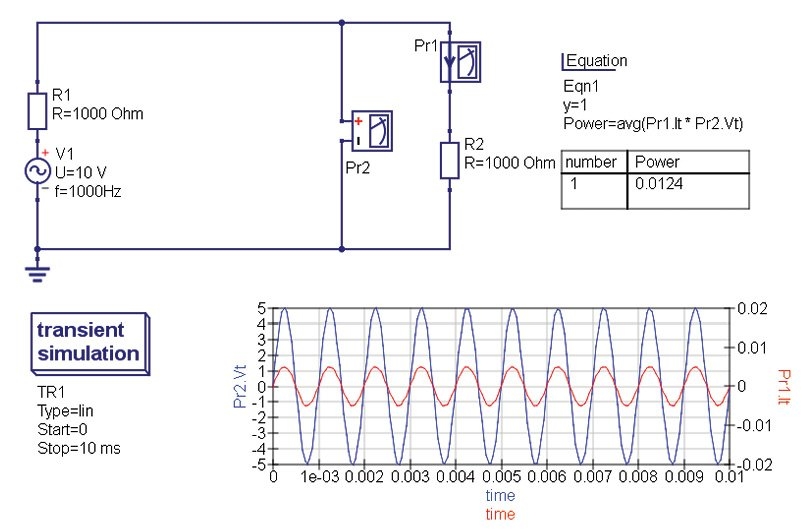FIGURE 2A source with a 1-kΩ impedance is perfectly matched with a 1-kΩ load. Output power is 12.4 mW in this case.
##### A MISMATCHED LOAD

OK, let’s keep the same source (1 kHz, 10 VPP, 1 kΩ), but connect it to a 4-Ω load. What happens? The simulation result is shown in Figure 3. The load has a far lower impedance than 1 kΩ, so the voltage drop across the internal resistance of the source becomes very high and the voltage applied on the load is just ±40 mV. The current through the circuit is ±10 mA, but the overall RMS power in the load is drastically reduced from 12.4 mW down to 0.197 mW.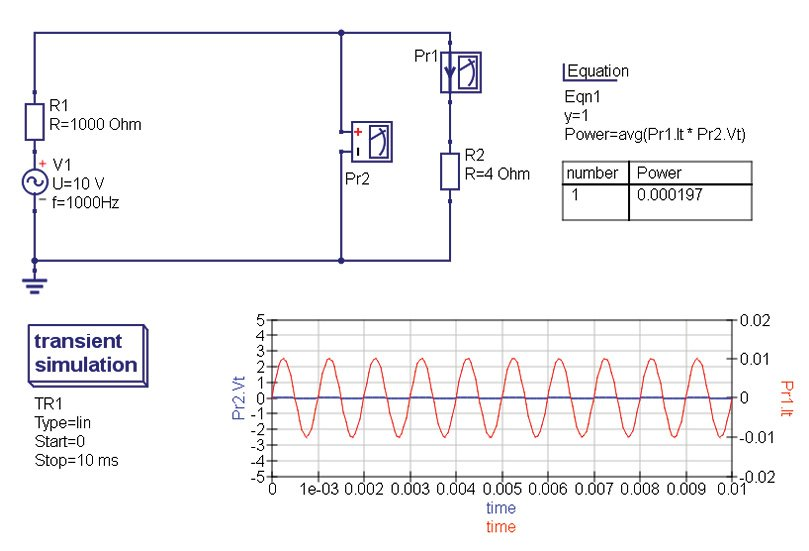FIGURE 3 When the load is heavily mismatched (here 1 kΩ to 4 Ω), the transferred power is very small.

This is a nice example of an impedance mismatch problem. You can reproduce it easily. Switch on your low-frequency generator, set it to 1 kHz (or use the audio output of your PC), add a 1-kΩ resistor to emulate a high-output impedance, and connect a 4-Ω loudspeaker. I’m sure you will have to come close to the speaker to hear anything.

Imagine that you can change neither the source’s 1-kΩ impedance nor the load’s 4-Ω impedance. How could you improve the situation (i.e., the energy transfer to the load)? By adding an impedance matching network between them. Such a network must transform a 1-kΩ impedance in a 4-Ω impedance. This must be done with as few extra losses as possible, so don’t even think of adding other resistors. Is it possible? Yes, indeed, and there are two main solutions.

The first is to simply use a transformer. Think about it. A high impedance means that voltages are high and currents are low, and this is the opposite for a low impedance. Therefore, in my example, you can use a transformer with more turns on the source side than on the load side. This will reduce the voltage and increase the current, and thus reduce the impedance. More exactly, the primary-to-secondary turn ratio must be the square root of the impedance ratio. In this case, it is 15.8 (i.e., √(1000/4)=15.8). You can try it by yourself using, for example, a small 230-to-15-V transformer, which has a turn ratio of 15.3 (i.e., 230/15). This should improve the audio level significantly.

A transformer is in fact a very good solution for impedance matching as it can work over a wide frequency range. It is indeed largely used for audio applications, ranging from tube amplifiers to microphones or high-power audio distribution networks. Suppose, however, that a transformer is too bulky or expensive for your application. Then the second solution would make sense. Using just two passive parts, either inductors or capacitors, and I will show you how in a minute. Theoretically, this second method is always possible, but it has two downsides. Firstly, the part values may not always be realistic, depending on the mismatch, and secondly such a passive matching network is narrowband by nature. This means it works only around a given frequency. Let’s see how we can use this method in my example.

The easiest way to calculate such a matching network is to use an online calculation tool. There is a nice one at www.leleivre.com (see Figure 4). Here I entered the design data: 1-kHz frequency, 1-kΩ source, and 4-Ω load. And then the tool proposed two LC networks. The first configuration uses a 2.5uF parallel capacitor (to ground) and a serial 10mH inductor. The second uses the opposite configuration. I selected the latter and added it to the simulation diagram (see Figure 5). You can double check. I kept a 1-kΩ resistor on the source side and a 4-Ω load. I then added L1 = 10 mH and C1 = 2.5 µH. The simulation shows that the power dissipated in the load is now increased from 0.197 to 12.4 mW. Remember, this is exactly we got with a perfectly matched 1-kΩ load!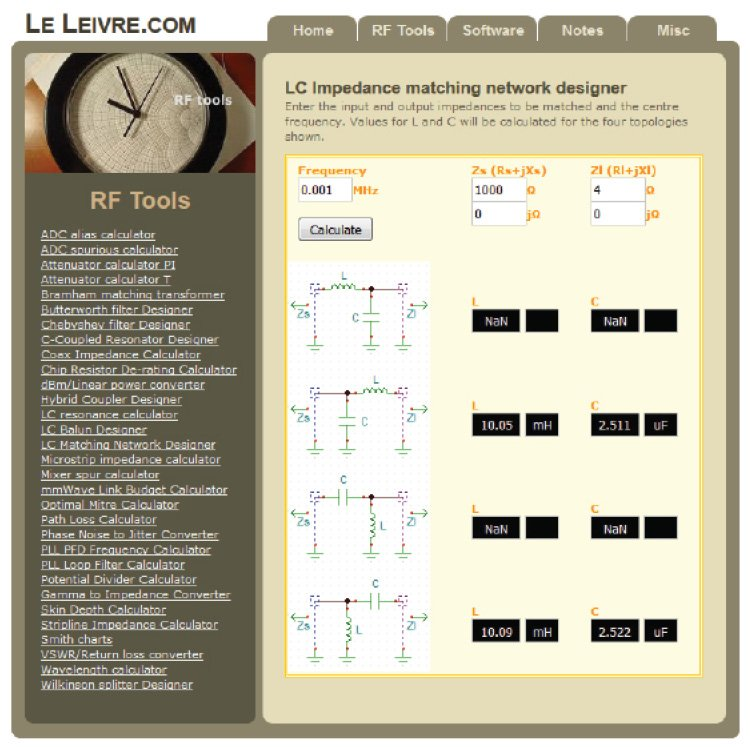FIGURE 4 An example of an online matching network calculator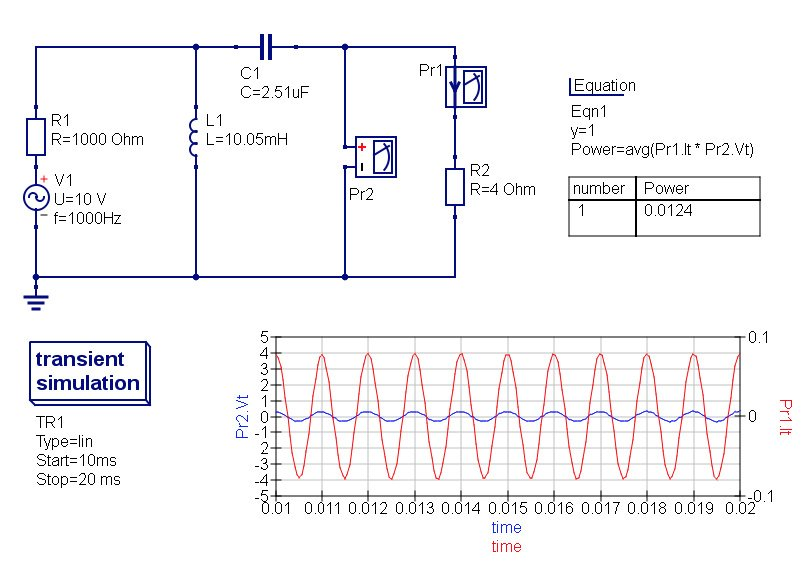FIGURE 5 With the addition of a LC matching network, the power transfer is back optimal (12.4 mW).

May be a quick manual calculation will help you to understand what’s going on. I’m sure you remember how to calculate the impedance of the inductor L1 and capacitor C1 at a frequency of 1kHz, right? We have:

If you consider the source resistor (R1) and the inductor (L1), they are in parallel. So their overall impedance is:

Do the math and you will get ZR1 + L1 = 4 + 63.4j. Look, the resistive part is now 4 Ω, as needed, but there is an inductive reactance of +63.4j. But, then, adding the serial capacitor cancels out this reactance as ZC1 = –63.4j, and we get 4 Ω. Yes!

##### LOW TO HIGH IMPEDANCE

Once again, there is nothing magic here. It’s just basic circuit behavior. If you build the test circuit with a generator, 1-kΩ resistor, and 4-Ω loudspeaker as I proposed, I strongly encourage you to grab a 10-mH inductor and a 2.5-µF capacitor (a 2.2 µF would very probably make the job). Add them to the circuit and you should get a far more audible signal from the speaker.

Thus far, I’ve demonstrated that a LC network can transform a high impedance in a lower one, but it also works the other way. On Figure 6, I took as an example a ±1-V source, still 1 kHz, with a 4-Ω internal resistance, and connected it to a 220-Ω load through the correct LC matching network, calculated using the same online tool. And, voila, the simulation shows that it works. The output power is 31.4 mW, which is the maximum possible. You can check it yourself. This may not seem strange, but look again at Figure 6 and focus on the waveforms. After the matching network, the voltage is nearly ±4-VPP. This is higher than the input voltage which was ±1 V. There is no power supply elsewhere. The circuit is fully passive. That means that such a low-to-high impedance network is actually increasing the signal voltage. This is very similar to a step-up voltage converter. The inductor and capacitor make a resonant network, which increase voltage. Of course, there is no power created by the circuit. The voltage is increased, but the current is reduced accordingly.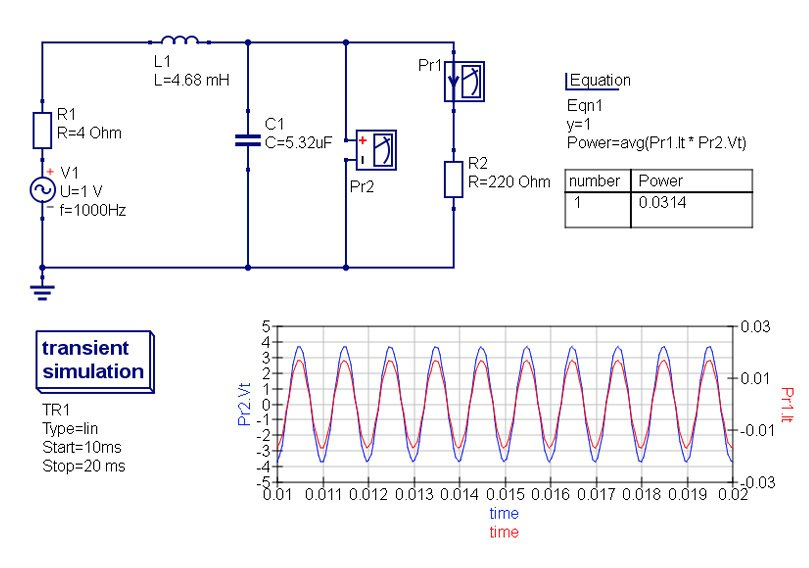FIGURE 6 An example of a low-to-high impedance matching network. The output voltage (in blue) is nearly four times higher than the source voltage.
##### BAND-PASS ISSUES

As explained, such an LC matching network works only at a single frequency. When I calculated the values of the parts, I entered “1 kHz” in the frequency box. What happens with the same network if the frequency of the input signal is no longer 1 kHz? I asked QUCS to calculate this for me (see Figure 7). As expected, the output power is maximal at a single frequency, here 1 kHz, and is lower as soon as the frequency is moving away from this value. More exactly, such a LC matching network has a quality factor Q, which sets the bandwidth of the matching. When the impedance mismatch gets higher, Q gets higher too, giving a narrower bandwidth.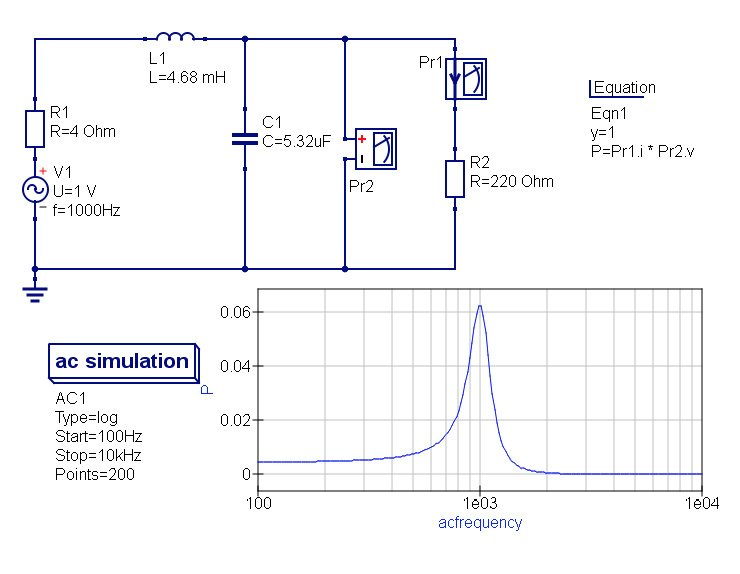FIGURE 7 The downside of an LC matching network is a quite narrow bandwidth.

If you read a book on impedance matching networks, like the very good one listed in the reference section, you will learn that more evolved matching networks can be build using not only two L or C’s but three. There parts can be arranged either in parallel/serial/parallel configuration (so-called pi network), or in serial/parallel/serial (T network). However, it can be shown that the simple LC network is in fact the one providing the wider frequency matching. All other pi or T networks will have a higher Q, so will be narrower in term of frequencies.

There are, however, techniques to get a wider impedance matching, but this requires more passive parts. The easiest is the chained LC method. As explained, a matching network has a wider frequency response if the input and output impedances are closer. So, rather then using one matching network to transform 4 Ω into 220 Ω, we could use an intermediate impedance Z, and transform firstly 4 ohm to Z, then Z to 220 Ω. As both will have a lower Q than a single network, the overall design will provide a wider bandwith. The optimal will be achieved with Z calculated as the geometric mean of input & output impedances, here 4 and 220 Ω, respectively. That gives Z ≈ 30 Ω (i.e., √800). I calculated two matching networks, from 4 to 30 Ω and then from 30 to 220 Ω. The simulation is provided in Figure 8. If you compare it to the previous versions, you will see that the overall output power is the same, but that the matching is far wider in term of frequencies. Of course, nothing prevents you from using more than two stages, you will get even wider matching. Don’t hesitate to download QUCS and to test it by yourself.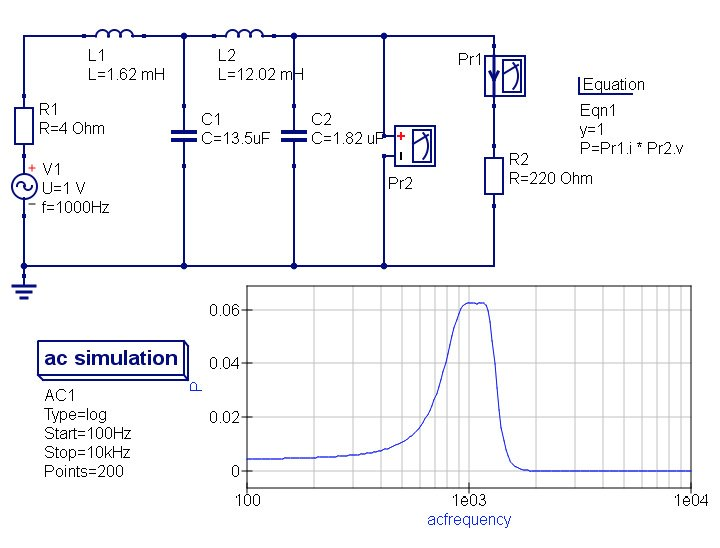FIGURE 8 Using a two-stage LC network increases the passband of the matching.
##### TRY YOURSELF

Here we are. In order to keep things as simple as possible, I only talked about purely resistive sources and loads, but the same calculations and methods can be used when there is a reactive load too, like an antenna. I also avoided presenting a great but intimidating tool—aka the Smith chart. This would be for another column.

Once again, you must be convinced that impedance matching is actually both useful and simple. And, as always, the best way to be convinced is to try it yourself. I hope that this article will help you for the first steps! Have fun with impedance matching!

RESOURCES
C. Bowick, J. Blyler, and C. Ajluni, “ Impedance Matching,” in J. S. Love, RF Front-End – World Class Designs, Newnes/Elsevier, 2009.
G. Breed, “Improving the Bandwidth of Simple Matching Networks,” 2008, High Frequency Electronicswww.highfrequencyelectronics.com/Mar08/HFE0308_Tutorial.pdf.

SOURCE
Quite Universal Circuit Simulator (QUCS)
QUCS Team | http://qucs.sourceforge.net/

PUBLISHED IN CIRCUIT CELLAR MAGAZINE • FEBRUARY 2017 #319 – Get a PDF of the issue

 Keep up-to-date with our FREE Weekly Newsletter! Don't miss out on upcoming issues of Circuit Cellar. Subscribe to Circuit Cellar Magazine Note: We’ve made the May 2020 issue of Circuit Cellar available as a free sample issue. In it, you’ll find a rich variety of the kinds of articles and information that exemplify a typical issue of the current magazine. Would you like to write for Circuit Cellar? We are always accepting articles/posts from the technical community. Get in touch with us and let's discuss your ideas.